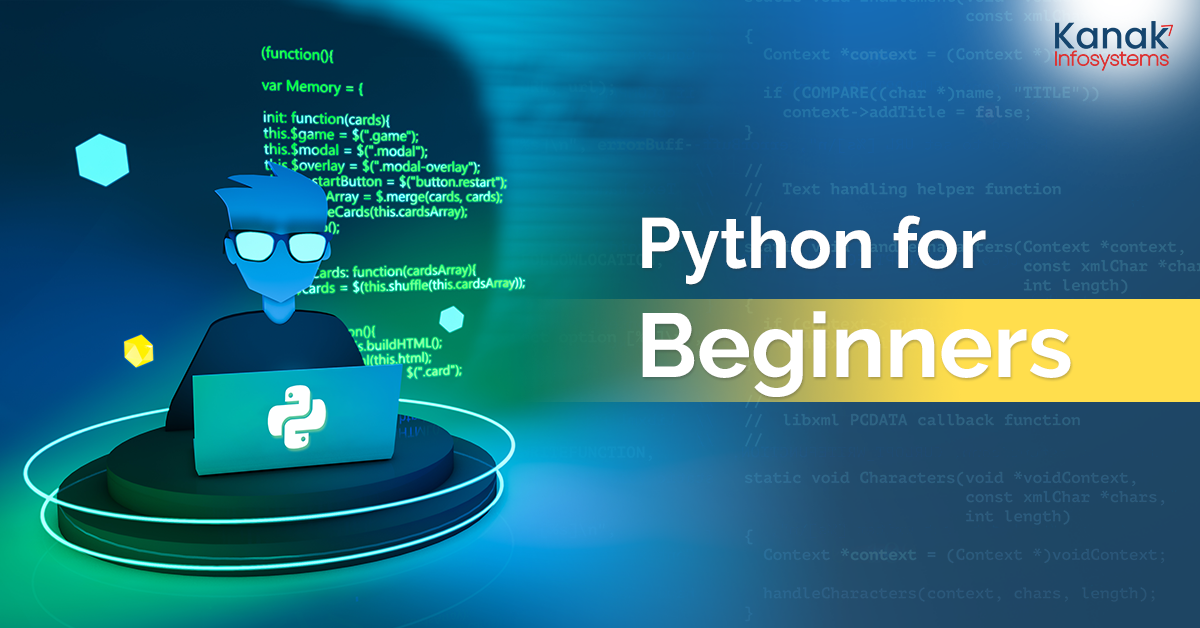Home Blog Python For Beginners# Python For Beginners

Python is an adaptable and easy to operate programming language that has garnered massive popularity in recent years. Doesn’t matter if you're just starting your journey as a programmer or looking to expand your skills, our blog will take you step- by- step through the fundamentals of Python with clear explanations and practical examples.

## Let's delve into the world of Python!

### 1. Installing Python:

Before deep diving, let's learn how to install Python on your computer.

### 2. Variables and Data Types:

In Python, you will be able to store and manipulate different types of data. You can also learn about variables, their naming conventions, and various data types such as integers, floating-point numbers, strings, booleans, and more. You can even explore how to assign values to variables and perform basic operations using arithmetic operators.

Master Python with insights delivered to your inbox. Subscribe today.

For Example:

``````For example:
# Variables and Data Types
age = 25
height = 1.75
name = "John"
is_student = True
``````
``````# Arithmetic Operations
sum_of_values = age + height
greeting = "Hello, " + name
print(sum_of_values)  # Output: 26.75
print(greeting)  # Output: Hello, John
``````

### 3. Control Flow and Conditional Statements:

You can learn how to control the flow of your program using conditional statements like if, elif, and else. You will understand how to use comparison operators (==, !=, >, <, >=, <=) and logical operators (and, or, not) to make decisions based on certain conditions.

For example:

``````# Control Flow and Conditional Statements
x = 5

if x > 10:
print("x is greater than 10")
elif x == 10:
print("x is equal to 10")
else:
print("x is less than 10")

# Output: x is less than 10
``````

### 4. Loops:

You can explore two fundamental loop structures in Python: the “for loop” and “the while” loop. You can easily learn how to iterate over a sequence of elements or perform a set of instructions repeatedly until a specific condition is met.

``````a.	For Loop
fruits = ["apple", "banana", "cherry"]
for fruit in fruits:
print(fruit)
# Output: apple
#         banana
#         cherry
``````
``````b.	While Loop
x = 0
while x < 5:
print(x)
x += 1
# Output: 0
#         1
#         2
#         3
#         4
``````

### 5. Functions:

Functions would allow you to break your code into reusable blocks. You will learn how to define functions, pass arguments, and return values. You will also understand the concept of scope and explore built-in functions and how to create your own custom functions.

``````# Functions
def greet(name):
return "Hello, " + name

result = greet("Alice")
print(result)  # Output: Hello, Alice
``````

### 6. Data Structures:

You will be discovering essential data structures in Python, such as lists, tuples, dictionaries, and sets. Along with learning how to manipulate and access elements within these structures.

``````# Data Structures
my_list = [1, 2, 3, 4, 5]
my_tuple = (1, 2, 3, 4, 5)
my_dict = {"name": "John", "age": 25}
my_set = {1, 2, 3, 4, 5}

print(my_list)  # Output: 1
print(my_tuple)  # Output: 3
print(my_dict["name"])  # Output: John
print(3 in my_set)  # Output: True
``````

### 7. Object-Oriented Programming (OOP)

#### 1. Classes and Objects:

Classes are the blueprints for creating objects, while objects are instances of a class. You will learn how to define classes, create objects, and access their attributes and methods.

``````# Classes and Objects
class Dog:
def __init__(self, name, age):
self.name = name
self.age = age

def bark(self):
print(f"{self.name} is barking!")

# Create an object of the Dog class
my_dog = Dog("Buddy", 3)
print(my_dog.name)  # Output: Buddy

my_dog.bark()  # Output: Buddy is barking!
``````

#### 2. Inheritance:

Inheritance allows you to create new classes based on existing ones, inheriting their attributes and methods. Understand how to establish parent-child relationships and leverage inheritance to reuse code and create specialized classes.

``````# Inheritance
class Animal:
def __init__(self, name):
self.name = name
def make_sound(self):
pass
class Cat(Animal):
def make_sound(self):
print("Meow!")
class Dog(Animal):
def make_sound(self):
print("Woof!")

my_cat = Cat("Whiskers")
my_cat.make_sound()  # Output: Meow!

my_dog = Dog("Buddy")
my_dog.make_sound()  # Output: Woof!
``````

#### 3. Encapsulation:

Encapsulation refers to the practice of bundling data and related methods within a class, hiding implementation details from the outside. Learn how to use access modifiers (public, private, protected) to control access to class members.

``````# Encapsulation
class Car:
def __init__(self):
self.__fuel = 100

def get_fuel(self):
return self.__fuel

def __update_fuel(self, value):
self.__fuel -= value

my_car = Car()
print(my_car.get_fuel())  # Output: 100
my_car.__update_fuel(20)  # Error: 'Car' object has no attribute '__update_fuel'
``````

#### 4. Polymorphism:

Polymorphism allows objects of different classes to be treated as interchangeable, based on a shared interface or superclass. Understand how to leverage polymorphism to write flexible and reusable code.

``````# Polymorphism
class Shape:
def area(self):
pass
class Rectangle(Shape):
def __init__(self, width, height):
self.width = width
self.height = height

def area(self):
return self.width * self.height

class Circle(Shape):
def area(self):
shapes = [Rectangle(4, 5), Circle(3)]
for shape in shapes:
print(shape.area())
# Output: 20
#         28.26
``````

To wrap it up, Python is a dynamic and user-friendly programming language which has garnered immense popularity over the past few years.

If you have any questions or queries, reach out to our experts now!